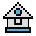Home

# EXP Function

#### Purpose:

To return e (the base of natural logarithms) to the power of x.

#### Syntax:

`EXP(x)`

x must be less than 88.02969.

If EXP overflows, the "Overflow" error message appears; machine infinity with the appropriate sign is supplied as the result, and execution continues.

EXP(x) is calculated in single precision, unless the /d switch is used when GW-BASIC is executed.

#### Examples:

```10 X = 5
20 PRINT EXP(X-1)
RUN
Ā54.59815```

Prints the value of e to the 4th power.